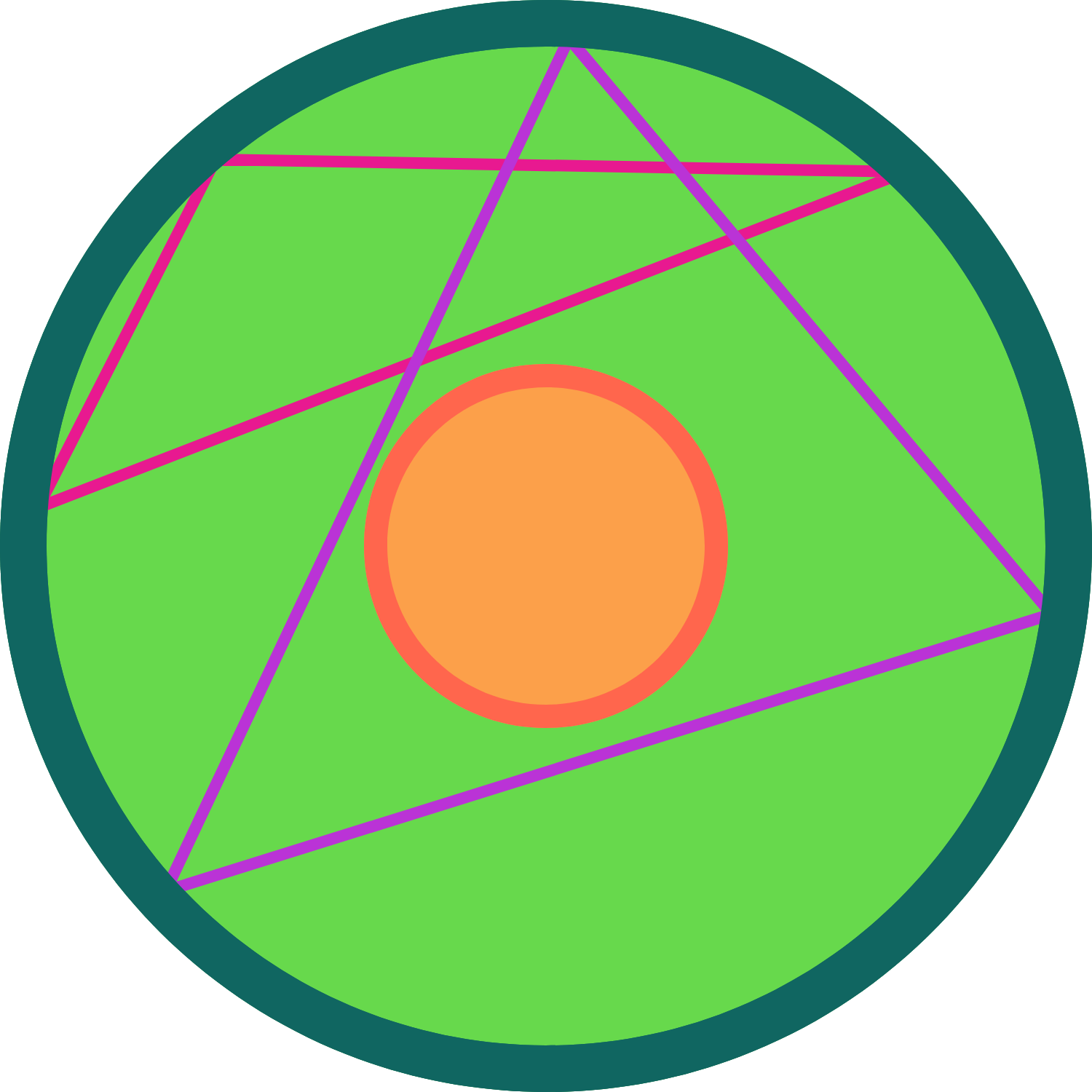# 1/3 is a nice number for this case

Probability Level 3Circles $L$ and $l$ share the same center. Circle $L$ has radius $3r$ and circle $l$ has radius $r.$ Points $A, B, C$ are chosen on the circumference of circle $L$ uniformly at random.

What is the probability that the centroid of $\triangle ABC$ lies in the interior of circle $l$?

×